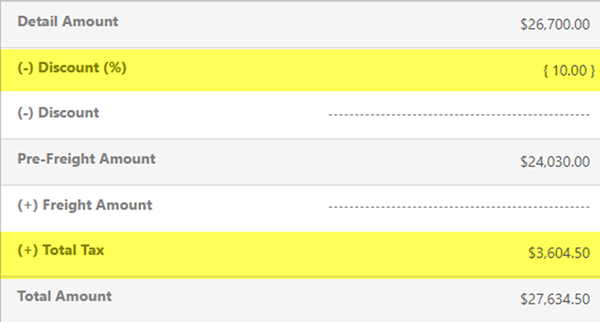# Tax and Discounts on Quotes/Orders in Dynamics 365 - Part 2 of 3

### Colin Maitland, 06 September 2018

In the second of this three-part series I will describe the first of two approaches that might be used for correctly calculating Total Tax on Quotes/Orders that have a Discount applied to the Quote/Order when it is desired that the calculation of Total Tax should take the Discount into consideration.

If there is no need for Tax to be applied at the Quote/Order Product level, then the following customisations might be used:

Step 1: Disable the Microsoft Dynamics 365 System Pricing Calculation. Refer to https://msdn.microsoft.com/en-us/library/dn817885.aspx. IMPORTANT NOTE: Disabling this option will require you to then provide custom programming to calculate all the previously system calculated pricing fields for Opportunities, Quotes Orders and Invoices.

Step 2: Remove the Tax field on the Quote/Order Product form. In this approach, this field will not be used by either the system or by Users.

Step 3: Override the system calculated Total Tax field on the Quote/Order to use one of the following calculations:

• If Total Tax includes tax on the Freight Amount, then Total Tax = Tax portion of (Pre-Freight Amount + Freight Amount); e.g. 15% of (\$24,030 + \$50) = \$3,612.50.

• If Total Tax does not include tax on the Freight Amount, then Total Tax = Tax portion of Pre-Freight Amount; e.g. 15% of \$24,030 = \$3,604.50.

e.g. when using Discount Percent …e.g. when using Discount Amount …In my next article I will describe a second approach that might be used.